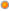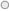# Q: My DAX formula in the PowePivot returns error message: "The value for columns "ColumnName1" in table "Table1" cannot be determined in the current context. How can I fix this?

User Rating: 4 / 5Q: My DAX formula in the PowePivot returns error message: "The value for columns "ColumnName1" in table "Table1" cannot be determined in the current context. How can I fix this?

A: You can get this error message in DAX when you are adding new calculated column or when you are adding new calculated measure.

If you are getting this error message when you are adding new calculated column, that means that you are referencing column "ColumnName1" from the table "Table1", but table where you adding this DAX calculated column is not related to the table "Table1". To fix this you need to create relationship between your table where you adding calculation and the table "Table1".

If you are getting this error when you are adding new calculated measure (in the PivotTable interface), that means that you are using Table1[ColumnName1] column in your DAX formula, but you did not specify what type of aggregation you want to use in formula context. To fix this you should specify column aggregation function, for example: SUM(Table1[ColumnName1]).

Tags: dax, faq, excel

2007-2015 VidasSoft Systems Inc.